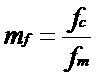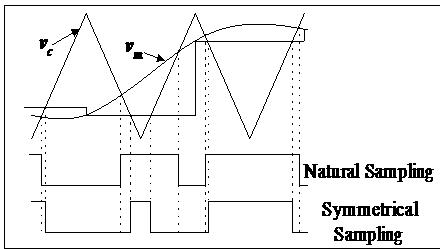SPWM modulation technique for multilevel inverter

By | December 12, 2015

Recently the multilevel inverter and converter have drawn tremendous interest for high voltage and high power application. The general structure of multilevel inverter and converter is to synthesize sinusoidal voltage waveform from several levels of voltages typically obtained from capacitor voltage source.

Low ratio of carrier frequency to modulation frequency is the best form of modulation for high power application, which is operating domain for multilevel inverter. For this purpose digital control of multilevel inverter using DSP/Microcontroller is preferred due to their dynamic controlling property. However, DSP/Microcontroller based system uses symmetrical regular sampling, asymmetrical regular sampling or resampling technique. These sampling techniques either introduces a delay in switching or requires a dedicated controller. In this work, a novel mathematical model of SPWM modulation technique for the multilevel inverter is presented which approaches the performance of traditional natural Sinusoidal Pulse Width Modulation (SPWM).

Introduction

The SPWM modulation technique is one of the most popular one used in wide range of industrial application where power switching converter is employed. Low ratio of the carrier frequency (fc) to modulation frequency (fm) is the best form of modulation for high power application. However, DSP/Micro-controller based system uses symmetrical regular sampling or resampling. These sampling techniques either introduces the delay in switching or requires a dedicated processor. This paper presents a simple mathematical model for SPWM technique. This technique is further extended and implemented on Artificial Neural Network (ANN).

Digital implementation of SPWM technique

Digital implementation of SPWM technique is based on classical SPWM technique with carriers and reference sine waveform. The only difference between them is, in digital SPWM a sine table consisting of values of sine waveform sampled at a certain frequency is used. As result reference waveform in digital SPWM represents a sample and hold waveform of the sine waveform.

This sampling of sine waveform comes in two variants;

1)      Symmetrical sampling.
2)      Asymmetrical sampling.

In symmetrical sampling, reference sine waveform is sampled at the only positive peak of the carrier waveform and the sample is held constant for the complete carrier period. This introduces the distortion in modulating signal and phase shift between modulating the signal and fundamental component of output voltage as shown in Fig 1. Here sampling frequency is equal to the carrier frequency. The phase shift is given by π/mf,where,  Fc = Carrier frequency.
Fm = Reference Sine wave frequency.Fig.1 Natural sampling versus symmetrical sampling

In asymmetrical sampling, the reference signal is sampled at positive as well as the negative peak of carrier frequency and held constant for half the carrier period. Here sampling frequency is twice the carrier frequency. Asymmetrical sampling is the preferred method since each switching edge is the result of the new sample and gives better performance as shown in Fig 2. The phase shift is by  π/2mfFig.2 Natural sampling versus asymmetrical sampling

Analog SPWM

Naturally sampled PWM is traditionally an analog technique where the input signal is naturally sampled by the carrier triangle waveform at the instant of the switching edge. Naturally sampled PWM can react instantly to changes in the input signal and produces no attenuation or distortion of the synthesized waveform. However as the ratio f1/fc rises for a given modulation depth M, the slew rate of f1 will exceed that of the carrier triangle and an extra pulse will be generated. If these additional pulses can be tolerated, the integrity of the synthesized waveform is preserved.

So for a multilevel, low switch frequency converter, a naturally sampled PWM modulator offers the most promise for wide bandwidth, low delay, and low distortion. Many multilevel converter implementations published in the literature demonstrate their modulation technique using natural sampled carrier based diagrams.

However natural sampled PWM is invariably implemented as an analog technique. An analog technique does not lend itself to a high power multilevel implementation. A digital implementation of analog SPWM is required which incorporate all desirable features of analog and digital SPWM. The first step toward this is Resampling method.

Resampling technique

Re-sampled uniform PWM is a digital implementation which approaches the frequency and transient response of natural PWM. Instead of sampling only once per switching edge at the beginning of the PWM switch period, further samples are taken during the switching period as shown in Fig 3. The position of the switch edge is determined by the most recent sample. This achieves the desired result, but some complications such as the possibilities of missed or multiple edges must be considered.

The Re-Sampling Ratio, rsr = fs/2fc is a measure of the rate of re-sampling (fs = Higher sampling frequency, fc = carrier frequency); only integer values ensure a sample is taken at the beginning of each switch period. Moderate values of the re-sampling ratio make a significant improvement in the frequency response, delay and distortion of the modulator, particularly for high modulation depth.Fig.3 Proposed Sinusoidal Pulse Width ModulationFig.4 Scheme for proposed SPWM method

Proposed SPWM for Multilevel Inverter

The allocation of proposed mathematical model can be extended to the multilevel inverter. The only difference in above procedure and procedure for determination ÃŽtk in the case of the multilevel inverter is that, as numbers of carriers are used in the multilevel inverter, an exact region of interaction of reference and carrier is to be known. In another word region of operation and transition from one region to another region should be determined.

Figure 5 shows the reference and carrier waveform arrangements necessary to achieve PD SPWM for a five-level inverter. Each shifted carrier is considered as one regionFig.5 – reference and carrier waveform in multilevel inverter

For example, for five level inverter region are r = 1, 0, -1, -2. Signals from region r = 1 are used to drive switch S1 and complementary signal are use to drive S1, similarly signal from region r = 0 are use to drive switch S2 and complementary signal are use to drive S2, Signal from region r = -1 are use to drive switch S3 and complementary signal are use to drive S3, signal from region r = -2 are use to drive switch S4 and complementary signal are use to drive S4.

The formula for finding time delay ‘ÃŽtk for positive and negative slop for different shifted carrier can be found by extending case for the two-level inverter.

The transition from one region of operation to the other can be decided on the basis of the calculated value of ‘ÃŽtk. To decide the transition from one region to other the criterion of transition for positive slop carrier cross-over is

(1)   If  ÃŽtk > 1/2fc

Then transition is from lower region to upper region, so r new = r old + 1 (where r = region)

(2)  If   ÃŽtk< 0

Then transition is from upper region to lower region, so r new = r old – 1

Similarly, to decide the transition from one region to other the criterion for negative slope carrier cross-over is

(1)   If ÃŽtk > 1/2fc

Then transition is formed upper region to lower region, so r new = r old 1 (where r = region)

(2)  If ÃŽtk < 0

Then transition is from lower region to upper region, so r new = r old + 1

Above procedure of calculating value of ÃŽtk can be surmised in flow-chart explain in Fig.6.Fig.6, Flowchart shows calculating a value of ÃŽtk

Artificial Neural Networks (ANN)

The science of artificial neural networks is based on the biological neuron. In order to understand the structure of artificial networks, the basic elements of the neuron should be understood. Neurons are the fundamental elements in the central nervous system.• The main advantage of neural networks is that it is possible to train a neural network to perform a particular function by adjusting the values of connections (weights) between elements.
• Neural networks are composed of elements operating in parallel. Parallel processing allows the increased speed of calculation compared to slower sequential processing.
• Artificial neural networks (ANN) have memory. In our case, a neural network controller could be trained to generate SPWM signal for multilevel inverter offline say in the Matlab (Simulink) environment. After training, the network weights are set. The ANN is placed in a feedback loop with the natural SPWM generator. The network will adapt the weights to improve performance as it generates SPWM signal.

Features of ANN

1)      Type of learning:

Neural networks have 3 main modes of operation supervised, reinforced and unsupervised learning.

2)      Neural Network Structures:

There are 3 main types of ANN structures -single layer feed-forward network, multi-layer feed-forward network and recurrent networks.

3)      Type of activation function (F):

There are 3 main types of activation function -tan-sigmoid, log-sigmoid and linear as shown in below figure. Different activation functions affect the performance of an ANN.Proposed SPWM technique for Multilevel Inverter using ANN

In proposed method, the neural network is used to determine the exact instant of the crossing of reference sine wave with carrier i.e. (tk+ÃŽtk), when input given to neural network is time instant tk as shown in Fig 4. The supervised learning method is used to train the neural network. In supervised learning, we have to specify the input-output target pair for training the neural network. Here input is given as time instants tk at which carrier waveform achieve their peaks (both positive and negative) and the corresponding target is specified by an exact instant of the crossing of carrier and reference i.e. (tk+ÃŽtk) for the corresponding value of tk.

Multilayer neural network having 3 layers is build. First two layers have 35 neurons with a transfer function as tan-sigmoid and the last layer has 1 neuron with a transfer function as a liner. Matlab package provides many power full tools for building and training the neural network.Author: Ravi Bandakkanavar

A Techie, Blogger, Web Designer, Programmer by passion who aspires to learn new Technologies every day. It has been 8 years since I have been publishing articles and enjoying every bit of it. I want to share the knowledge and build a great community with people like you.

Suggested articles for you:

One thought on “SPWM modulation technique for multilevel inverter”

1.Amruth

This is a nice article. Can you post a sinetable for 5 level inverter ? I’m still not clear how to control each IGBT from DSP controller in multi-level inverter